# 「javaScript 」从地基开始打造一座万丈高楼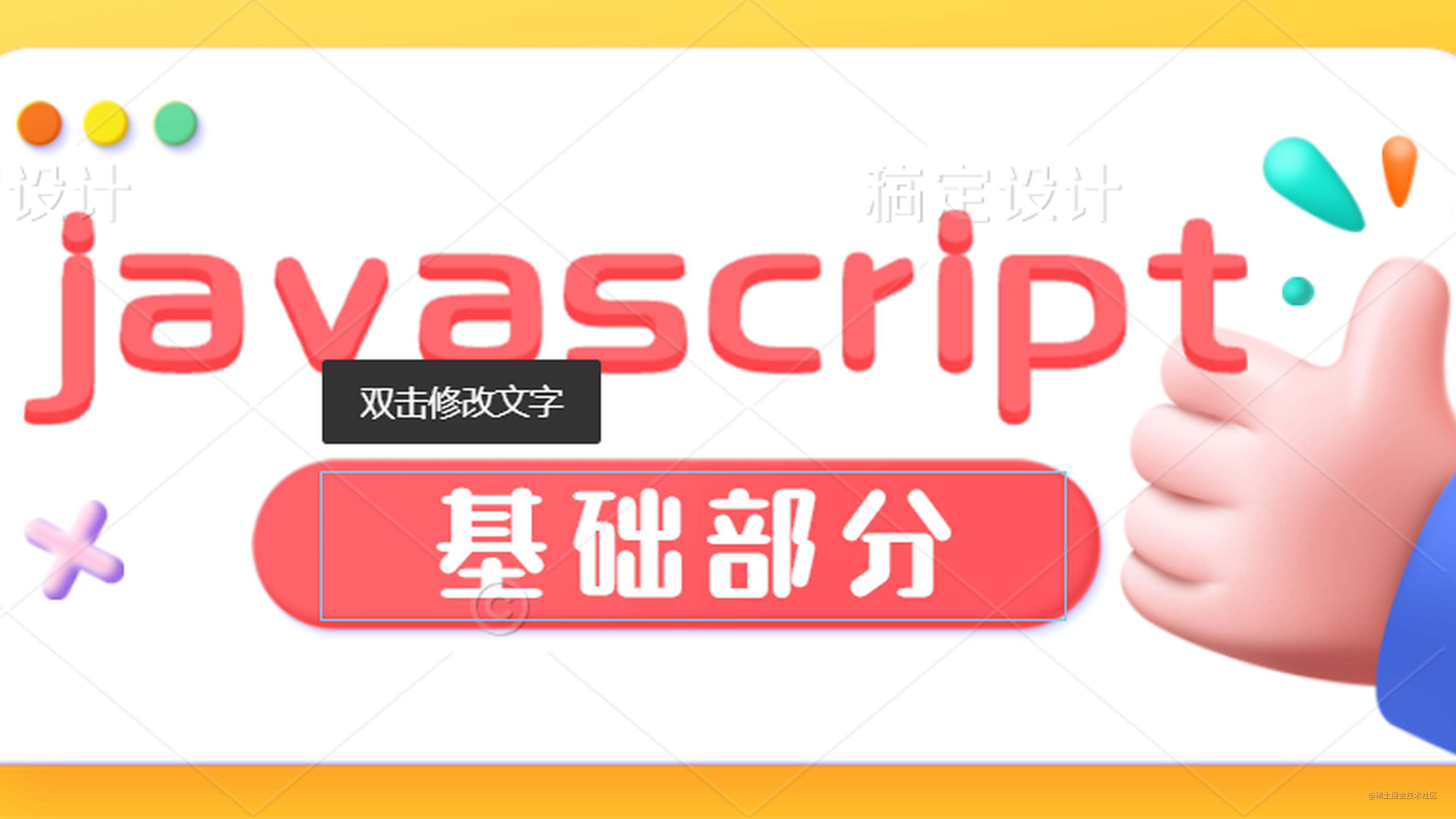## 前言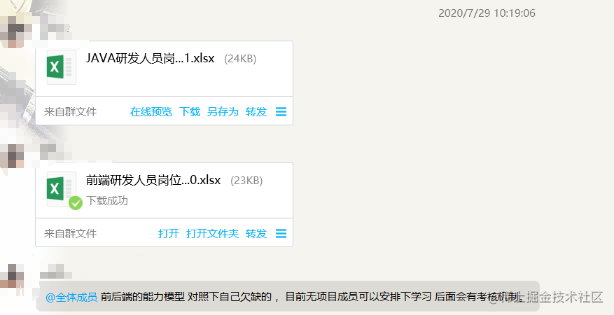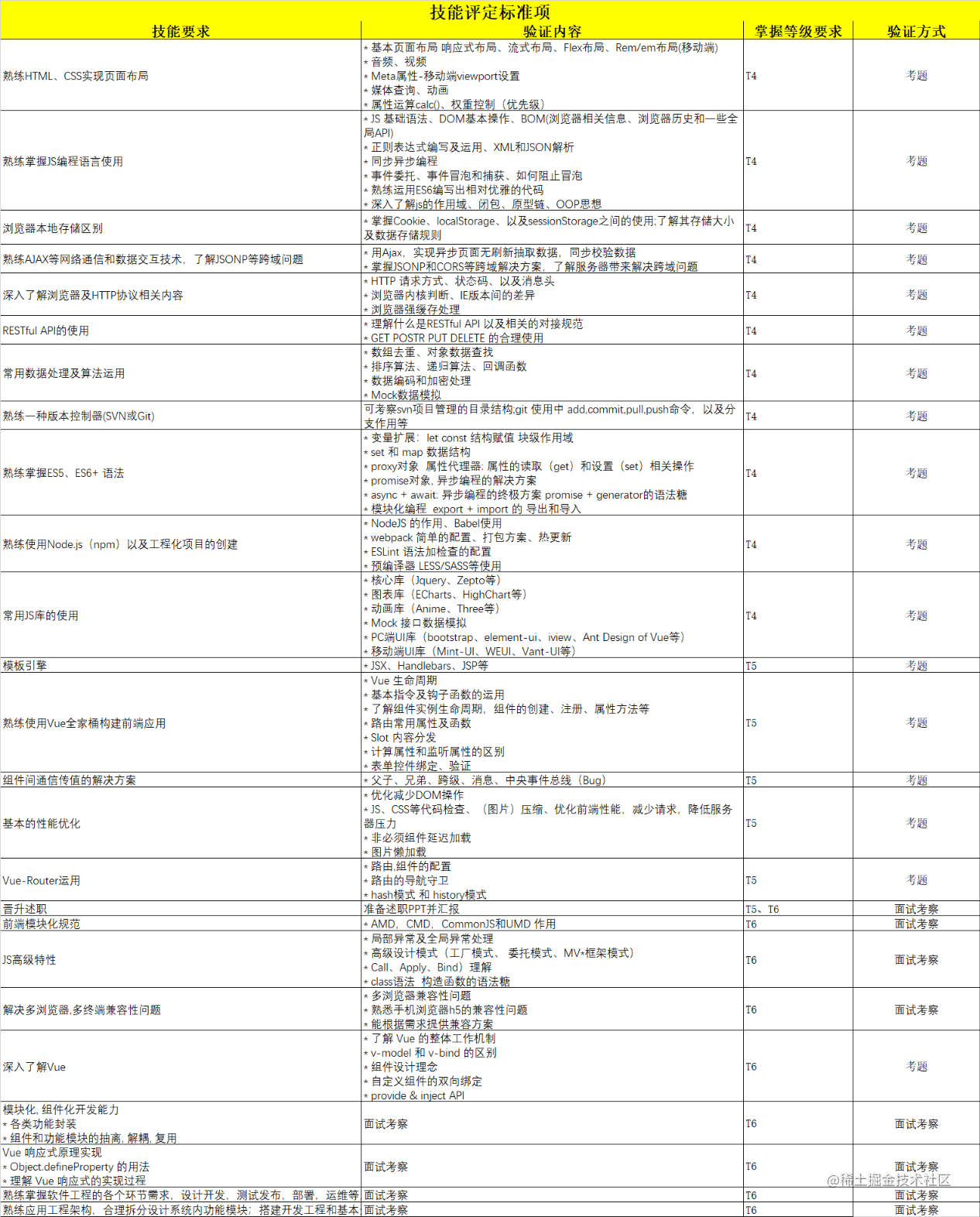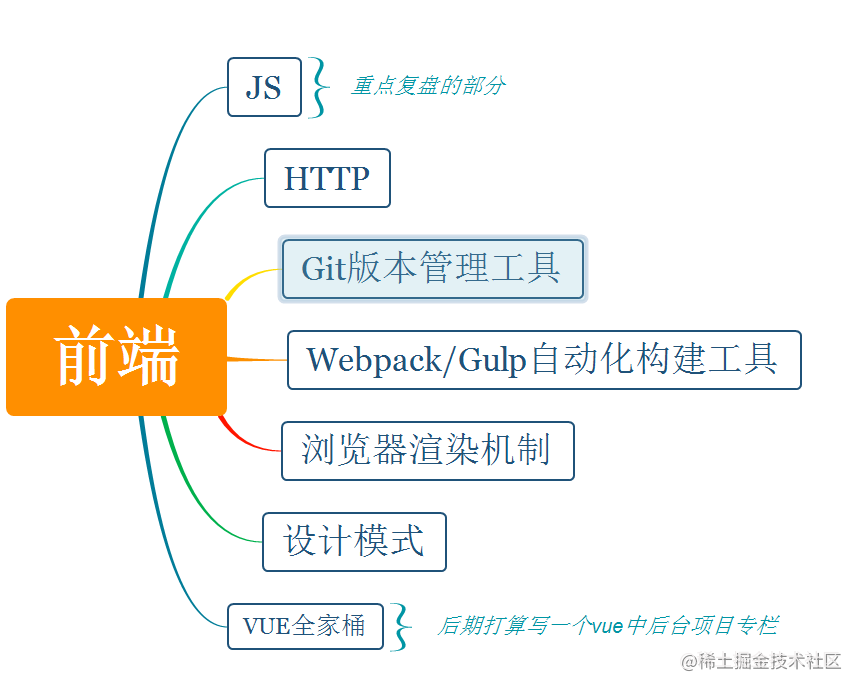## 转变

![](cdn.jsdelivr.net/gh/it-beige… 」从地基开始打造一座万丈高楼/前端体系.png)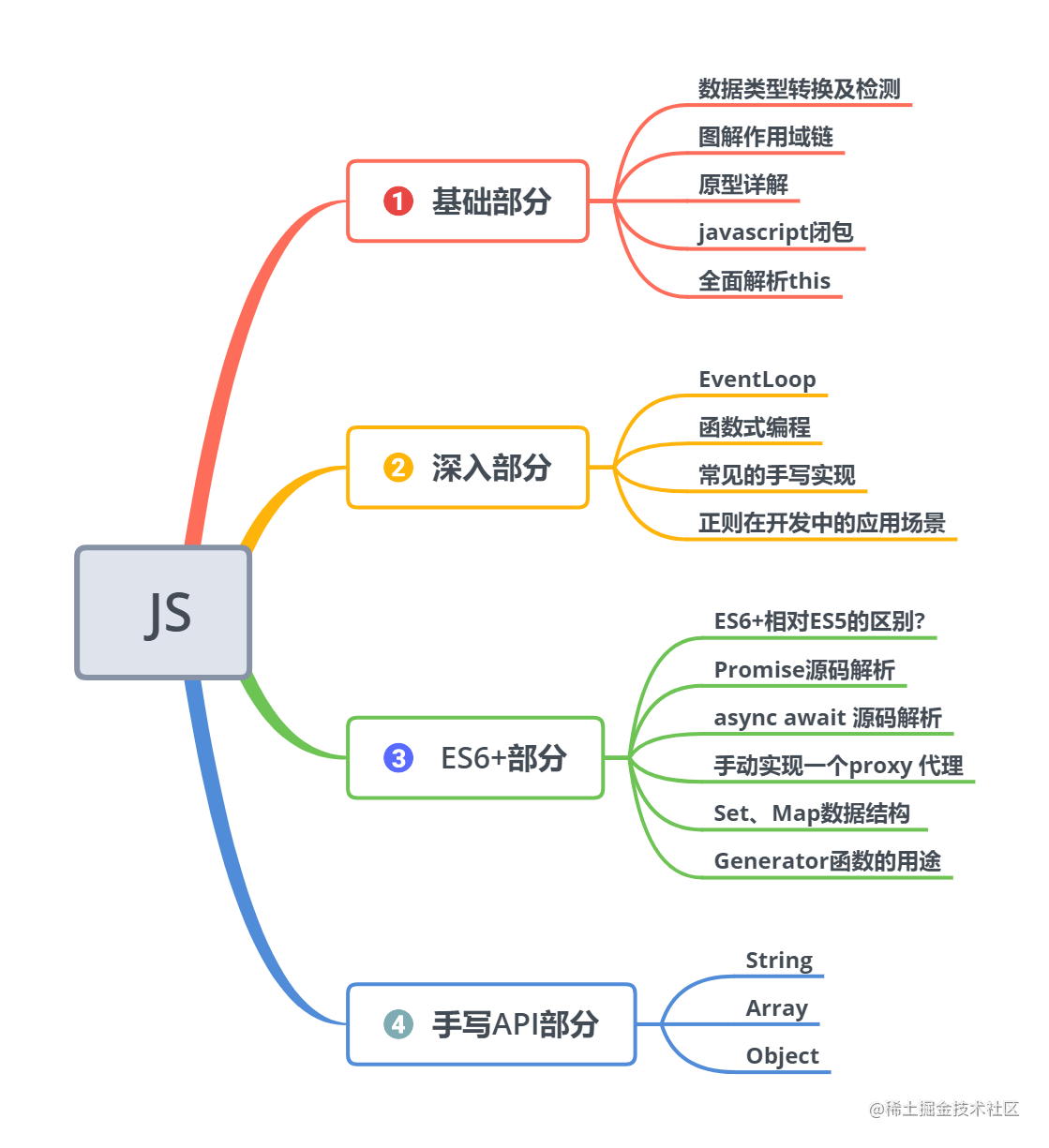# 数据类型的检测及转换

• 基本数据类型(值类型)
• Number
• String
• Boolean
• Null
• Undefined(js中独有的空)
• Symbol (ES6新增)
• BigInt (ES6新增)
• 引用数据类型(引用类型)
• Object
• 普通对象
• 函数对象
• 数组对象
• 正则对象
• 日期对象
• ....

## 数据类型检测

• typeof
• instanceof
• constructor
• Object.prototype.toString.call() 【最佳的方式】

### typeof

``````let a = '北歌',
b = 18,
c = [12, 23],
d = {a: 1},
e = new Map(),
f = new Set(),
g = new RegExp('A-Z'),
h = new Function(),
i = null,
j = undefined,
k = Symbol(),
l = false;

console.log(typeof a); // String
console.log(typeof b); // Number
console.log(typeof c); // object
console.log(typeof d); // object
console.log(typeof e); // object
console.log(typeof f); // object
console.log(typeof g); // object
console.log(typeof h); // function
console.log(typeof i); // object
console.log(typeof j); // undefined
console.log(typeof k); // symbol
console.log(typeof l); // boolean
// => 问题: 不能很好的区分数组和对象

`typeof` 可以检测的数据类型有几种？？究竟哪几种自己数。

`问题一`

``````console.log(typeof []) ?
console.log(typeof typeof []) ?

`答案`

`问题二`

``````function foo() {
let a = b = 0;
a++;
return a;
}

foo();
typeof a; // => ???
typeof b; // => ???

`答案`

`foo()`作用域或全局作用域中都没有声明变量 `b`。因此JavaScript将表达式 `b = 0` 解释为 `window.b = 0`

``````function foo() {
let a;  window.b = 0;  a = window.b;  a++;
return a;
}

foo();
typeof a;        // => 'undefined'
typeof window.b; // => 'number'

typeof a是 'undefined'。变量a仅在 foo()范围内声明，在外部范围内不可用。
typeof b等于'number'。b是一个值为 0的全局变量

### instanceof

instanceof运算符用来检测一个实例对象在其原型上是否存在一个构造函数的prototype属性

``````a instaceof B
// => 用来检测 a 是否是 B 的实例, 是为true, 否则反之

[] instanceof Array; // true
{} instanceof Object; // true
new Date() instanceof Date; // true
new RegExp() instanceof RegExp // true

``````console.log(1 instanceof Number) // false
console.log(new Number(1) instanceof Number)// true

``````let arr = [1, 2, 3];
console.log(arr instanceof Array) // true
console.log(arr instanceof Object);  // true
function fn() {}
console.log(fn instanceof Function) // true
console.log(fn instanceof Object) // true

// => 原理就是判断右边的prototype是否在左边的原型链上, 这就导致了在类的继承中检测的结果不正确
let arr = new Array('1'),
fun = new Function();

console.log(arr instanceof Function); // false

arr.__proto__.__proto__ = Function.prototype;
console.log(arr instanceof Function); // true
// 下面简单的画了个图(有点丑小伙伴们能看懂就行)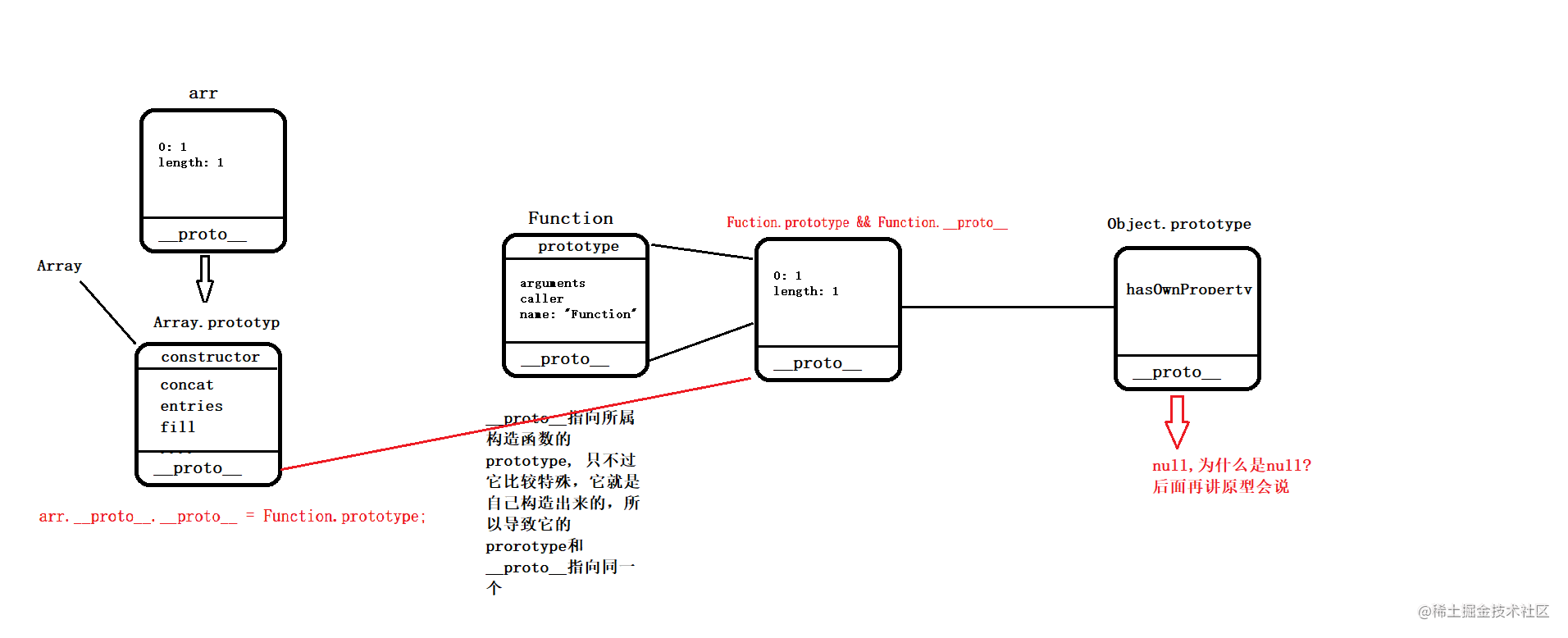instaceof原理

``````function myInstanceof(leftVal, rightVal) {
let proto = leftVal.__proto__,
rightPrototype = rightVal.prototype;
while(true) {
if (proto === null) return false;
if (proto === rightPrototype) return true
proto = proto.__proto__;
}
}

``````function Foo(){}
function BFoo(){}
Foo.prototype = new BFoo();
let foo = new Foo();
console.log(foo instanceof Foo); ?
console.log(foo instanceof BFoo); ?

``````console.log(String instanceof String);
console.log(Object instanceof Object);
console.log(Function instanceof Function);
console.log(Function instanceof Object);

function Foo(){}
function BFoo(){}
Foo.prototype = new BFoo();
console.log(Foo instanceof Function);
console.log(Foo instanceof Foo);• 所有实例对象都会有一个`__proto__`（我们叫它原型链属性）指向所属构造函数的`prototype` （原型属性）
• 浏览器会给每个构造函数开辟一个`prototype`（原型对象), 该对象提供了供实例对象调用的成员属性和方法
• 每个`prototype`都会自带一个`constructor`指回了该原型对象所属的构造函数（重写了原型对象会造成`constructor`丢失）
• 内置类中最大的Boss不是Object基类，Object也是通过内置Function构造出来的，所以`Object.__proto __`=== `Function.prototype`
• 内置Function也是通过自身构造出来，所以`Function.__proto__ ` === `Fcuntion.prototype`

``````/**
* 内置类:
*  - Function
*  - Object
*  - Number
*  - Array
*  - String
*  - Boolean
*  - RegExp
*  - Date
*  - Map
*  - Set
*  .......
*/

console.log(String.__proto__ === Function.prototype);
console.log(Number.__proto__ === Function.prototype);
console.log(Boolean.__proto__ === Function.prototype);
console.log(Date.__proto__ === Function.prototype);
console.log(RegExp.__proto__ === Function.prototype);
console.log(Object.__proto__ === Function.prototype);
console.log(Array.__proto__ === Function.prototype);
console.log(Map.__proto__ === Function.prototype);
console.log(Set.__proto__ === Function.prototype);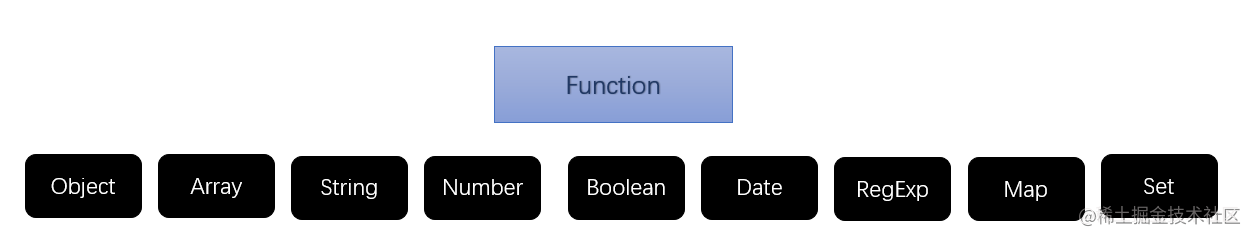``````console.log(Object instanceof Object);
console.log(Foo instanceof Foo);

L = `Object.__proto__ `= `Function.prototype` R = `Object.prototype`

L = `Foo.__proto`__ = `Funtion.protoype` R = `Foo.prototype` (重写为了BFoo的实例对象)

### constructor

``````function Foo(){};
var foo = new Foo();
// 其原理就是找该实例对象的的原型链对象中的constructor

`问题一`

`问题二`

``````function Fn(){}
Fn.prototype = new Array()
var f = new Fn
console.log(f.constructor) // Array

Fn.prototype = {}
console.log(f.constructor) // Object

### Object.prototype.toString.call()

• 返回某个数据的内部属性`[[class]]`，能够帮助我们准确的判断出某个数据类型

``````let a = '北歌',
b = 18,
c = [12, 23],
d = {a: 1},
e = new Map(),
f = new Set(),
g = new RegExp('A-Z'),
h = new Function(),
i = null,
j = undefined,
k = Symbol(),
l = false;

console.log(Object.prototype.toString.call(a)); // [object String]
console.log(Object.prototype.toString.call(b)); // [object Number]
console.log(Object.prototype.toString.call(c)); // [object Array]
console.log(Object.prototype.toString.call(d)); // [object Object]
console.log(Object.prototype.toString.call(e)); // [object Map]
console.log(Object.prototype.toString.call(f)); // [object Set]
console.log(Object.prototype.toString.call(g)); // [object RegExp]
console.log(Object.prototype.toString.call(h)); // [object Function]
console.log(Object.prototype.toString.call(i)); // [object Null]
console.log(Object.prototype.toString.call(j)); // [object Undefined]
console.log(Object.prototype.toString.call(k)); // [object Symbol]
console.log(Object.prototype.toString.call(l)); // [object Boolean]

``````let isType = (type) => (o) => Object.prototype.toString.call(o) === `[object \${type}]`;
console.log(isType('Array')([]));

`Symbol.toStringTag`公知的符号是在创建对象的默认字符串描述中使用的字符串值属性。它由该`Object.prototype.toString()`方法在内部访问。大体意思就是说这个方法决定了刚刚我们提到所有数据类型中`[[class]]`这个内部属性是什么。

``````let map = new Map(),
set = new Set();
console.dir(map);
console.dir(set);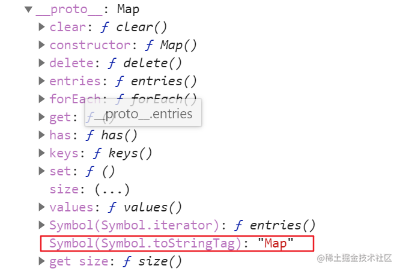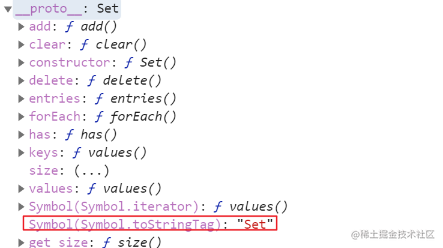``````console.log(map.toString()); // [object Map]
console.log(set.toString()); // [object Set]
console.log(arr.toString()); // '12, 23'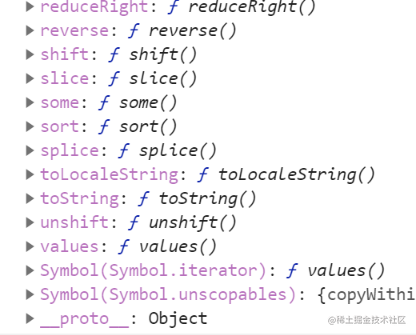• 没有`Symbol.toStringTag`内置属性的类型在调用`toString()`的时候相当于是`String(obj)`这样调用转换为相应的字符串
• `Symbol.toStringTag`内置属性的类型在调用`toString()`的时候会返回相应的标签(也就是`"[object Map]"`这样的字符串)

``````class Super {}
console.log(Object.prototype.toString.call(new Super()))

``````class Super {
get [Symbol.toStringTag]() {
return 'Test'; // => Symbol.toStringTag允许我们自定义返回的类标签
}
}
console.log(Object.prototype.toString.call(new Super())); // [object Test]
// 注意: Symbol.toStringTag重写的是Super这个类的实例对象的标签， 而不是重写Super这个类的标签
console.log(Object.prototype.toString.call(Super)) // "[object Function]"

• typeof
• 不能细分对象和数组
• 对于null这种特殊对象也不能区分
• instanceof
• 对于字面量创建的基本类型不能很好的判断
• 对于实例的判断只要左侧在其原型链上的对象和右侧原型对象一致就行，在类继承上会出现问题
• 对于null和undefined这类型不能检测
• constructor
• 对于null和undefined无效
• 对于constructor这种方式， 如果原型对象重写或继承也会出现问题## 数据类型转换

### 强制转换

#### 其他的数据类型转换为String

• toString()方法
• String()函数

``````let a = 123,
b = null,
c = undefined;
a.toString() // "123"
b.toString() // "报错"
c.toString() // "报错"

// => toString()
String({a: 1}) // "[object Object]" => 不管啥对象都是转换成这个
String([1, 2, 3]) // "1,2,3"
String() // "1"
String(null) // 'null'
String(undefined) // 'undefined'
String(new Map()) // "[object Map]"
String(new Set()) // "[object Set]"

// => 特殊情况
console.log(class A {}.toString()) // 'class A {}'
console.log(function () {}.toString()) // 'function () {}'
console.log(/(\[|\])/g.toString()) // '/(\[|\])/g'
console.log(new Date().toString()) // 'Fri Mar 27 2020 12:33:16 GMT+0800 (中国标准时间)'

#### 其他的数据类型转换为Number

• Number()函数
• parseInt()&parseFloat()
• +, - , * / 操作

Number()函数对于基本类型转换，null false 转换为0， true转换为1。

``````// 基本类型
Number(true) // 1
Number(false) // 0
Number(null) // 0
Number(3.15) // 3.15
Numer(0x12) // 18 => 可以识别 hex dec oct bin 进制

• parsetInt() 把一个字符串(如果不是先转换为字符串)中的整数部分解析出来
• parseFloat() 把一个字符串中小数(浮点数)部分解析出来
``````parseInt(null) // => NaN

// 出一道很典型的面试题考下大家
let arr = [10.18, 0, 10, 25, 23, 345, 2345]
arr = arr.map(parseInt)
// arr => ?

``````/*
parseInt([value], [n])
- value: 把value转换为数字,先将value转换为String, 然后中String左侧第一个字符查找, 把找到的有效数字字符转换为数字,直到遇到一个非有效数字字符为止
- n: 把value做n进制的数据, 最后转换为十进制

- 一般情况下都看做10, 但默认并不是10。比如：字段以0x |0X开头，就是走16进制转换
- n范围[2~36] 不在这个之间的，除了0和10一样，剩下都是NaN
*/

``````/**
* parseInt('10.18', 0)
* => 看作10进制： 10
* parseInt('0', 1)
* => 不在范围：NaN
* parseInt('10', 2)
* => 看作2进制： '10' 再把它转换为1十进制
* => 二进制转10进制： 用当前数(0) * 当前进制(2)的当前数的[n](0)次幂
* => 0：  0 * 2^0 => 0 * 1 = 0
* => 1:   1 * 2^1 => 1 * 2 = 2
* => 结果 2
*
* parseInt('25', 3)
* => 看作3进制: 包含0，1，2
* => 2 * 3^0 => 2 * 1
* 结果  2

* parseInt('23', 4)
* => 看作4进制: 包含0，1，2, 3
* => 3 * 4^0 => 3 * 1
* => 2 * 4^1 => 2 * 4
* => 结果 11
*
*
* parseInt('345', 5)
* => 看作5进制: 包含0，1，2, 3, 4
* => 4 * 5^0 => 4 * 1
* => 3 * 5^1 => 3 * 5
* => 结果 19
*
* parseInt('2345', 6)
* => 看作6进制: 包含0，1，2, 3, 4, 5
* => 5 * 6^0 => 5 * 1 => 5
* => 4 * 6^1 => 4 * 6 =>  24
* => 3 * 6^2 => 3 * (6*6) => 108
* => 2 * 6^3 => 2 * (6*6*6) => 432
* => 结果 569
*
*/

#### 其他的数据类型转换为Boolean

• Boolean
• ! (转换为布尔类型再取反)
• !! (转换为布尔类型取反再取反，相当于没变只是转换为布尔类型了)

null, undefined, 0, NaN, ''(空字符串)这五个转换布尔为false其余都会true, 记住这个规则就行

#### 引用类型转换为其他类型

• 对象转换为字符串
• 对象转换为数值

• 调用对象的toString()方法, 如果返回的是原始类型, 将值转换为字符串并返回
• 如果没有toString()这个方法或返回的不是原始类型, 调用valueOf()方法获取它的原始类型值, 将值转换为字符串返回
• 如果对象两个方法都没有或都没有转换成功直接抛错

• 调用对象的valueOf()方法获取它的原始类型, 将值转换为数值返回
• 如果没有valueOf()这个方法或返回的不是原始类型, 调用toStrig()方法获取它的原始类型值, 将值转换为数值返回
• 如果对象两个方法都没有或都没有转换成功直接抛错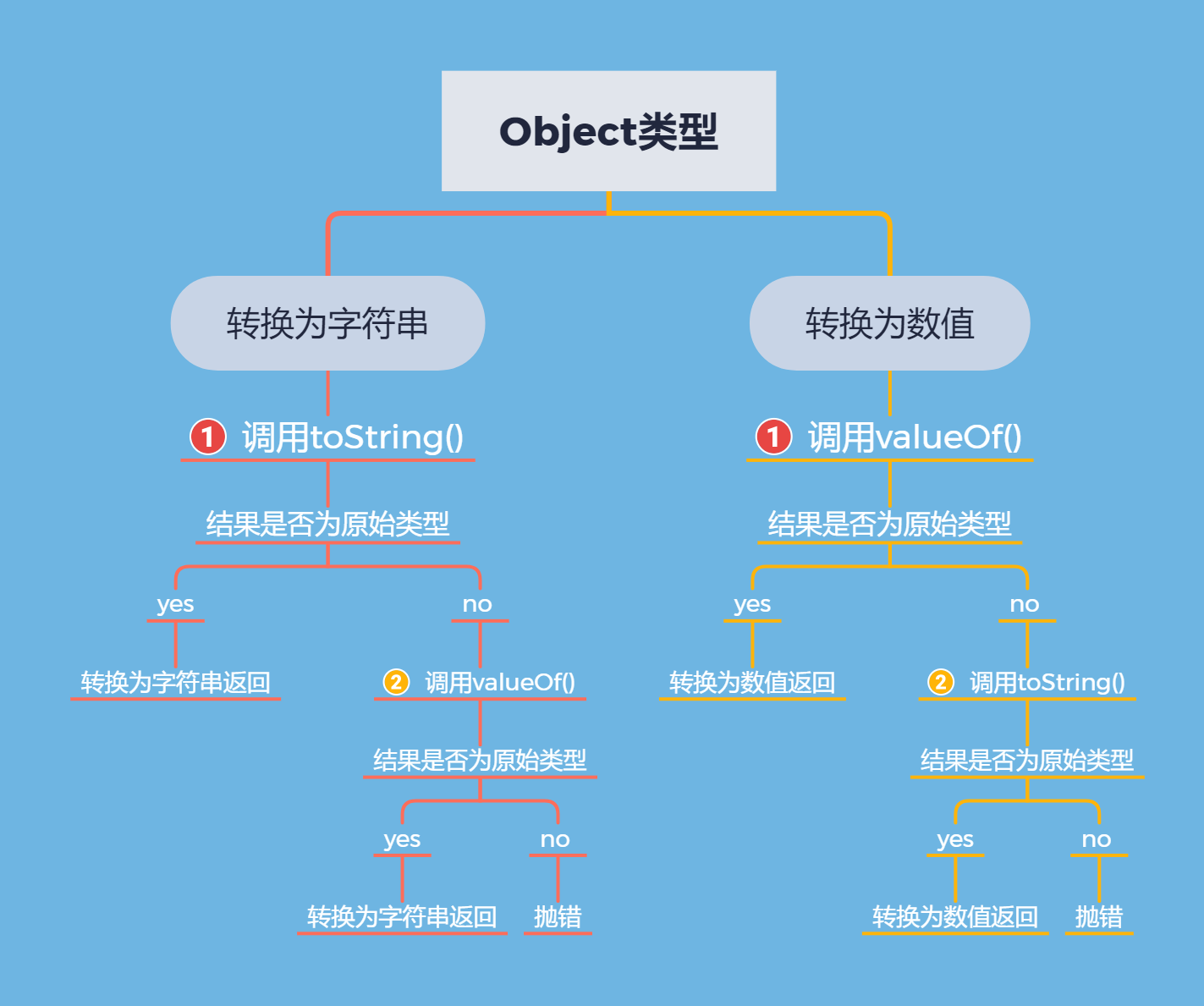``````[] => ''
 => 1
[12, 23] => '12, 23'
[' '] => ' '
{a: 1} => '[object Object]'

``````let obj = {}, // 用于转换数值
arr = [], // 用于转换为字符串
testError = {};

// ===================================> 转换字符串
obj.toString = function() { // 先走它
return  // 返回的不是原始类型, 意味着要继续向后走了
}
obj.valueOf = function() {
return 12 // 返回了原始值12, 注意是Number类型,
}

console.log(String(obj), typeof String(obj)); // 12 string => 最后将结果转换为字符串返回了

// ===================================> 转换数值
arr.toString = function() {
return '12' // 返回了原始值12, 注意是String类型,
}
arr.valueOf = function() { // 先走它
return {}  // 返回的不是原始类型, 意味着要继续向后走了
}
console.log(Number(obj), typeof Number(obj)); // 12 number => 最后将结果转换为数值返回了

// ===================================> 最后再来一个走不通的
testError.toString = function() {
return {}
}
testError.valueOf = function() {
return {}
}
console.log(Number(testError)); // 抛错

// 其他流程大家可以自己测试一下

``````let a = ?
if (a == 1 && a == 2 & a == 3) {
console.log(1)
}
// 怎么样可以让结果输入1???

``````let a = {value: 0}
a.valueOf = function() { // 我们通过重写valueOf在每次比较的时候调用valueOf让a的自增
return ++this.value;
}
if (a == 1 && a == 2 & a == 3) { // 在比较的时候调用valueOf获取对象的原始值
console.log(1)
}

// 除了重写valueOf方法也可以通过重写toString方法达到上面一样的效果
let a = {
value: 0,
toString: function() {
return ++this.value;
}
}

``````// => Object.definedProperty()创建对象某个属性时，通过get再获取属性时做操作， 代码如下：
let value = 0;
Object.defineProperty(window, 'a', {
get() {
console.log('调用了');
return ++value;
}
})

if (a == 1 && a == 2 & a == 3) {
console.log(1)
}

### 隐式转换

1. 当使用 `==``&&``||` 等逻辑操作符进行判断时
2. 当使用 `+ - * /` 四则运算符进行操作时

#### 自动转换为布尔值

``````if (12 + 'px') {
// 12 + 'px => Boolean('12px') 为true
}

#### 自动转换为数值

``````// 记住一点除了 + 运算符如果有一端出现字符串就是拼接，其他都是跟数学一样的运算
true + 1 // 2
'true' + 1 // 'true1'
2 + null // 2
undefined + 1 // NaN
2 + NaN // NaN 任何值和NaN做运算都得NaN
'5' - '2' // 3
'5' * '2' // 10
true - 1  // 0
'1' - 1   // 0
'5' * []    // 0
false / '5' // 0
'abc' - 1   // NaN

#### 自动转换为字符串

``````'6' + true // "6true"
'6' + false // "6alse"
'6' + {} // "6object Object]"
'6' + [] // "6 => '6' + String([]) => '6' + ''
'6' + function (){} // "6function (){}"
'6' + undefined // "6undefined"
'6' + null // "6null"

• "=="比较中的隐式转换
``````{name: '北歌'} == {name: '北歌'} // => 对于引用类型比较的是内存地址
[] == [] => false

let obj1 = {},
obj2 = obj1;
obj1 == obj2 // true

• 两端都是不同类型比较，都是转换为数值类型再比较，唯一的区别是（对象 == 字符串 ）将对象转换为字符串
• null == undefined 为true 全等为false，需要特殊记忆
• NaN和谁都不相等，对于NaN的比较可以用ES6中的Object.is()方法

``````12+true+false+null+undefined+[]+'北歌'+null+undefined+[]+true
!!" " + !!"" - !!false ||document.write("能打印嘛")

``````console.log([] == 0);
console.log(![] == 0);
console.log([] == ![])
console.log([] == []);
console.log({} == !{});
console.log({} == {});``````console.log(![] == 0);

• 首先规则大家应该知道，不同类型比较都转换数字
• ![] 使用"!"强制转换为布尔类型再取反 false
• false == 0 false转数字为0
• 0 == 0

#### 一道输出为sb的答案解析

「2021.5.11补 」

``````(!(~+[])+{})[--[~+""][+[]]*[~+[]] + ~~!+[]]+({}+[])[[~!+[]]*~+[]]

• 运算符优先级
• ~(按位操作符)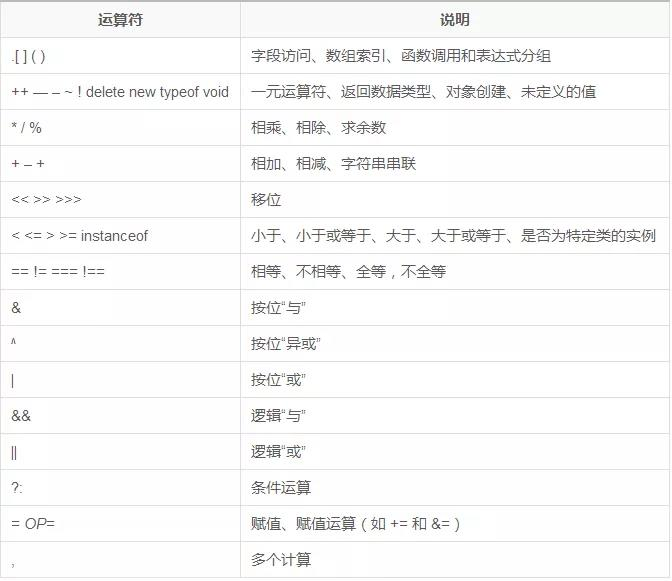``````[1, 2] => 1 、// 成员访问
{} + [] => [] => // 数组

``````~0 => 0取反 -> -0(js本质中是没有-0一说的) => 0 - 1 => -1
~1 => 1取反 -> -1 => -1 - 1 => -2
~-1 => -1取反 -> 1 => 1 - 1 => 0

「 解析图」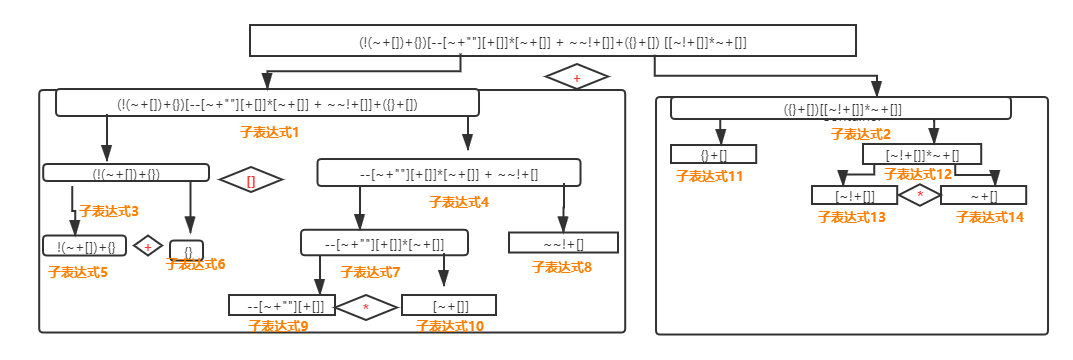「 解析代码」

``````1(子表达式1)                                     2(子表达式2)
(!(~+[])+{})[--[~+""][+[]]*[~+[]]~~!+[]]  +    ({}+[])[[~!+[]]*~+[]]

「 子表达式1 」部分

``````1.1(子表达式3)
(!(~+[])+{})
[]
1.2(子表达式4)
--[~+""][+[]]*[~+[]] + ~~!+[]

1.1.1(子表达式5)
!(~+[])+{}
+
1.1.21(子表达式6)
{}

1.2.1(子表达式7)
--[~+""][+[]]*[~+[]]
+
1.2.2(子表达式8)
~~!+[]

1.2.1.1(子表达式9)
--[~+""][+[]]
*
1.2.1.2(子表达式10)
[~+[]]

「 子表达式2 」部分

``````2.1(子表达式11)
{}+[]
[]
2.2(子表达式12)
[~!+[]]*~+[]]

2.2.1(子表达式13)
[~!+[]]
*
2.2.2(子表达式14)
~+[]

「 整体运算 」

``````(!(~+[])+{}) => (!(-1)+{}) => 'false[object Object]'

``````{}
- ({}) 被括号包裹着的解析为对象
- {}  解析为代码块
~+[] 这种叠加运算符一定是就近原则

``````(子表达式4)  2 + 1 => 3
--[~+""][+[]]*[~+[]] + ~~!+[]

(子表达式7) -2 * [-1] => 2
--[~+""][+[]]*[~+[]]

--[~+""][+[]] => --[-1] => -2
*
[~+[]] => [-1]

(子表达式8) 1
~~!+[] => ~~true => ~-2 => 1

``````({}+[])   [[~!+[]]*~+[]]

``````({}+[]) => '[object Object]'
[[~!+[]]] * ~+[] => [[-2] * -1] =>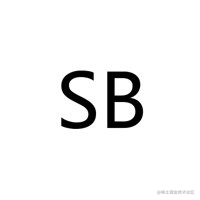# 图解作用域链

#### 引言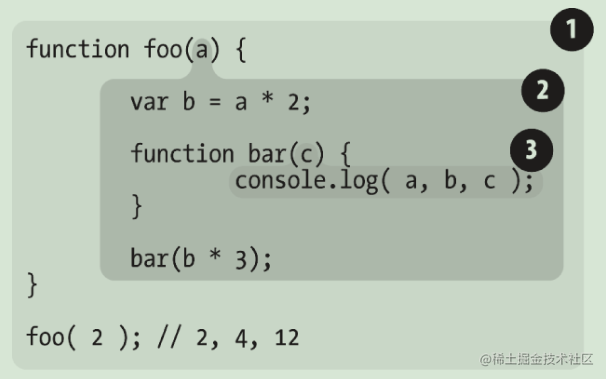• 泡泡1是全局作用域，有标识符foo；
• 泡泡2是作用域foo，有标识符a, b, bar；foo函数中的形参a也相当于函数中的私有变量(标识符)
• 泡泡3是作用域bar，仅有标识符c。

#### 作用域 (scope)

• 全局作用域：拥有全局作用域的对象可以在代码的任何地方访问到。
• 局部作用域：和全局作用于相反，局部作用域一般只能在固定代码片段内可以访问到。最常见的就是函数作用域
##### 全局作用域

1. 最外层的函数以及最外层变量:
``````var globleVariable= 'global';  // 最外层变量
function globalFunc(){         // 最外层函数
var childVariable = 'global_child';  //函数内变量
function childFunc(){        // 内层函数
console.log(childVariable);
}
console.log(globleVariable)
}
console.log(globleVariable);  // global
globalFunc();                 // global
console.log(childVariable)   // childVariable is not defined
console.log(childFunc)       // childFunc is not defined

1. 未定义直接赋值的变量(由于变量提升使之成为全局变量)
``````function func1(){
special = 'special_variable'; // 没有用var声明自动提升全局变量
var normal = 'normal_variable';
}
func1();
console.log(special);    //special_variable
console.log(normal)     // normal is not defined

// 有var和不带var有什么区别呢？？
// => 带var不能被delete删除

var a = 10;
b = 20;
delete a; // false 删除不了这个变量存储的值
delete b; // true 可以删除

``````// 变量冲突
var globleVariable = "person";
let globleVariable = "animal"; // Error, thing has already been declared

``````// 张三写的代码
var name = 'beige'

// 李四写的代码
var name = 'yizhan'
console.log(name);  // yizhan

##### 局部作用域

1、函数作用域

``````function test1() {
var a = 10;
console.log(a);
}

function test2() {
var a = 20;
console.log(a);
}

test1(); // 10
test2(); // 20

// => 两个函数内的同名变量a相互独立，互不影响。

2、块级作用域

ES6 引入了块级作用域，让变量的生命周期更加可控，块级作用域可通过新增命令let和const声明，所声明的变量在指定块的作用域外无法被访问。块级作用域在如下情况被创建：

1. 在一个函数内部
2. 在一个代码块（由一对花括号包裹）内部

let声明和var声明的区别：

1. 不存在变量提升
2. 不允许重复声明
4. 不存在和全局window之间的相互映射

`代码演示`

``````// 变量提升
console.log(str); // undefined;
var str = '北歌';

// 不存在变量提升
console.log(str); // str is not defined;
let str = '北歌';

// 允许重复声明  => 后面覆盖前面
var a = 10;
var a = 20;

// 不允许重复声明 => Identifier 'b' has already been declared
let a = 10;
let a = 20;

// TDZ
function foo1() {
console.log(a); // a is not defined
var a = 10;
}

function foo2() {
console.log(a); // Cannot access 'a' before initialization
let a = 10;
}

foo1()
foo2()

// 存在映射
var a = 10;
console.log(window.a); // 10;
window.a = 20;
console.log(a); // 20

// 不存在映射
var a = 10;
console.log(window.a); // undefined => window对象没有这个属性
window.a = 20;
console.log(a); // 10

``````for (let i = 0; i < 10; i++) {
// ...
}
console.log(i);
// ReferenceError: i is not defined

``````var a = [];
for (var i = 0; i < 10; i++) {
a[i] = function () {
console.log(i);
};
}
a(); // 10

``````var a = [];
for (let i = 0; i < 10; i++) {
a[i] = function () {
console.log(i);
};
}
a(); // 6

``````for (let i = 0; i < 3; i++) {
let i = 'abc';
console.log(i);
}
// abc
// abc
// abc

``````{
// 父作用域
let i;
for (i = 0; i < 3; i++) {
// 子作用域
let i = 'abc';
console.log(i);
}
}

#### 作用域链

##### JavaScript是如何执行的？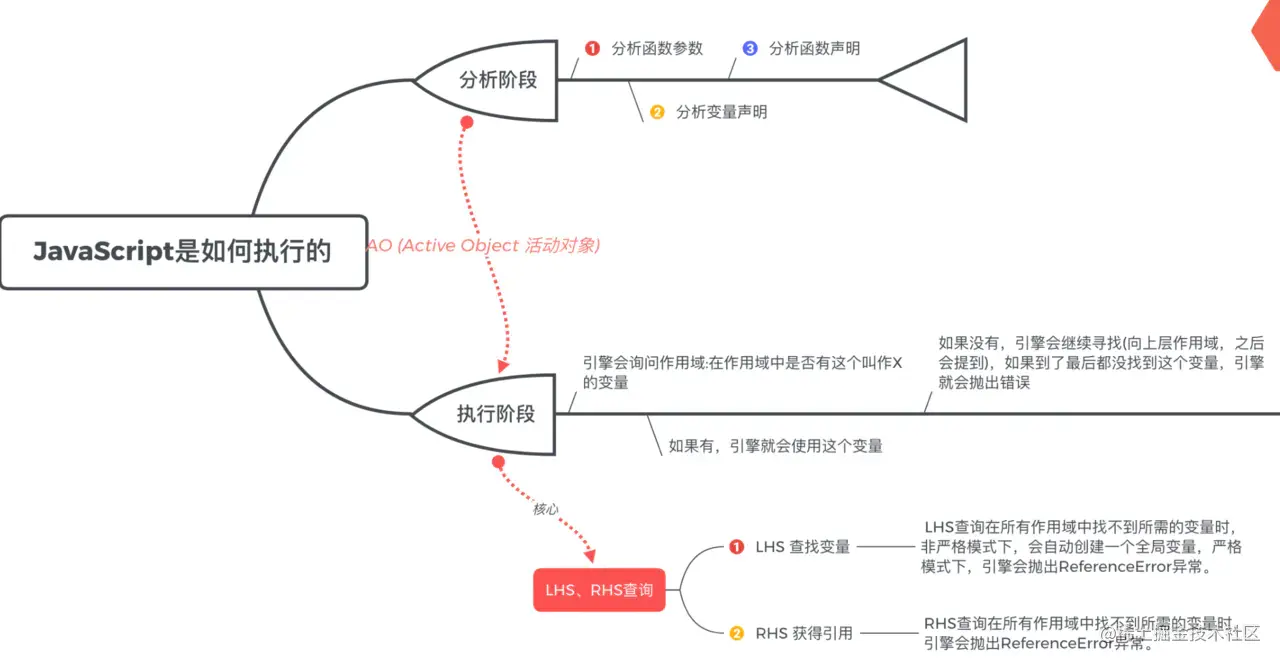• 引擎 •从头到尾负责整个 JavaScript 程序的编译及执行过程。
• 编译器 •引擎的好朋友之一，负责语法分析及代码生成等脏活累活
• 作用域 • 引擎的另一位好朋友，负责收集并维护由所有声明的标识符（变量）组成的一系列查 询，并实施一套非常严格的规则，确定当前执行的代码对这些标识符的访问权限。

JavaScript代码执行分为两个阶段：

###### 分析阶段

javascript编译器编译完成，生成代码后进行分析

• 分析函数参数
• 分析变量声明
• 分析函数声明

###### 执行阶段

• 引擎询问作用域，作用域中是否有这个叫X的标识(变量)
• 如果作用域有标识(变量)，引擎会使用这个标识(变量)
• 如果作用域中没有，引擎会继续寻找（向上层作用域），如果到了最后都没有找到这个标识(变量)，引擎会抛出错误。

JavaScript执行举例说明

``````function a(age) {
console.log(age);
var age = 20
console.log(age);
function age() {
}
console.log(age);
}
a(18);

``````AO = {}

``````形参：AO.age = undefined

``````// 第3行代码有var age
// 但此前第一步中已有AO.age = 18, 有同名属性,不做任何事

``````// 第5行代码有函数age
// 则将function age(){}付给AO.age
AO.age = function age() {}

``````AO.age = function age() {}
//之后
AO.age=20
//之后
AO.age=20

``````function age(){

}
20
20

`找`过程中LHS和RHS查询特殊说明

LHS，RHS 这两个术语就是出现在引擎对标识(变量)进行查询的时候。在`《你不知道的javaScript(上)》`也有很清楚的描述。`freecodecamp`上面的回答形容的很好：

LHS = 变量赋值或写入内存。想象为将文本文件保存到硬盘中。 RHS = 变量查找或从内存中读取。想象为从硬盘打开文本文件。

#### LHS和RHS特性

• 都会在所有作用域中查询
• 严格模式下，找不到所需的变量时，引擎都会抛出`ReferenceError`异常。
• 非严格模式下，`LHR`稍微比较特殊: 会自动创建一个全局变量
• 查询成功时，如果对变量的值进行不合理的操作，比如：对一个非函数类型的值进行函数调用，引擎会抛出`TypeError`异常

LHS和RHS举例说明，例子来自于《你不知道的Javascript(上)》

``````function foo(a) {
var b = a;
return a + b;
}
var c = foo( 2 );

``````引擎：我说作用域，我需要为 c 进行 LHS引用, 你见过吗?

``````c=, a=2(隐式变量分配), b=

RHS(读取内存)

``````读foo(2), = a, a ,b
(return a + b 时需要查找a和b)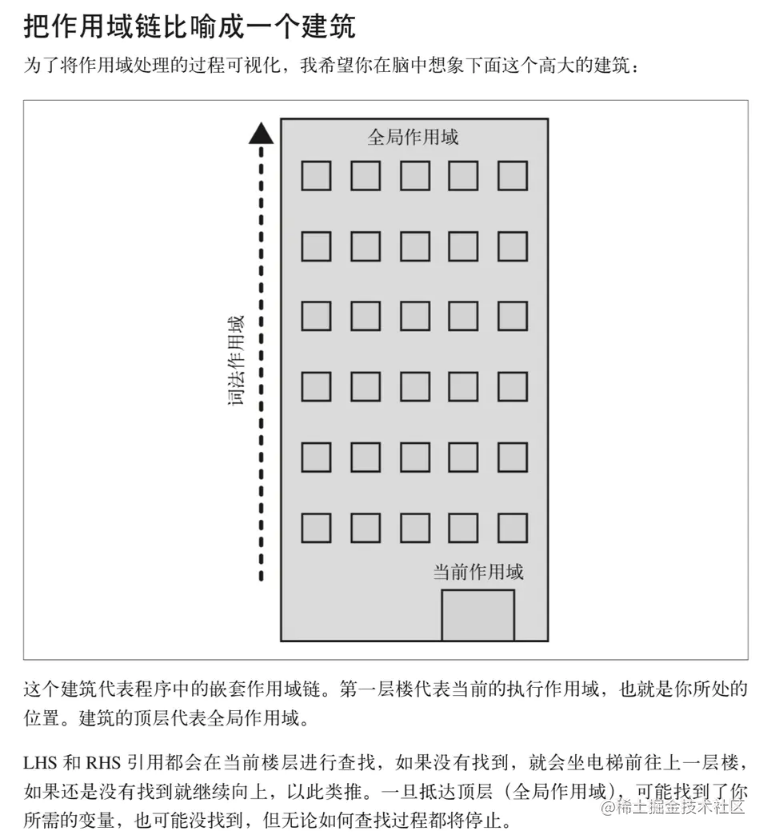``````let str = 'global' // 全局作用域
function outer() { // 第二层作用域
let str = 'outer';
return function inner() { // 第一层作用域
console.log(str);
}
}
let inner = outer();
inner(); // outer

# 原型详解

• 所有实例对象都会有一个`__proto__`（我们叫它原型链属性）指向所属构造函数的`prototype` （原型属性）
• 浏览器会给每个构造函数开辟一个`prototype`（原型对象), 该对象提供了供实例对象调用的成员属性和方法
• 每个`prototype`都会自带一个`constructor`（构造器属性）指回了该原型对象所属的构造函数（重写了原型对象会造成`constructor`丢失）
• 内置类中最大的Boss不是Object基类，Object也是通过内置Function构造出来的，所以`Object.__proto __`=== `Function.prototype`
• 内置Function也是通过自身构造出来，所以`Function.__proto__ ` === `Fcuntion.prototype`

## ES5和ES6之后的不同

### 写法上的不同

``````// ===========================> ES5的写法
// 将父类原型指向子类
function inheritPrototype(subType, SuperType) {
let prototype = Object.create(SuperType.prototype); // 获取父类的原型副本
prototype.constructor = subType; // 解决子类重写原型对象constructor丢失问题
subType.prototype = prototype
}

// 父类
function Es5Super(colors) {
this.colors = ['red', 'blue', 'green'];
}
Es5Super.prototype.getColor = function (index) {
console.log(this.colors[index]);
}

// 子类
function Es5(name) {
this.name = name;
}

inheritPrototype(Es5, Es5Super);

// 在原型上写方法
Es5.prototype.getName = function() {
console.log(this.name + '公有成员');
}
// 在函数对象上添加成员
Es5.getName = function() {
console.log(this.name + '私有成员');
}

// ===========================> ES6的写法
class ECMAScript{
constructor(version) {
this.version = version;
}
}

class Es6 extends ECMAScript {
constructor(name, version) {
super(version)
this.name = name;
this.version = this.version;
}

getName() { // 在原型上写方法
console.log(this.name + '公有成员');
}

getVersion() {
console.log(this.version);
}

static getName() { // 在函数对象上添加成员
console.log(this.name + '私有成员');
}

}

let es5 = new Es5('es5')
let es6 = new Es6('es6', 'ES2015')

console.log(es5);
console.log(es6);
// => 在ES6出现类继承之后基本就大一统了继承。

## 从原型神图中说说自己的理解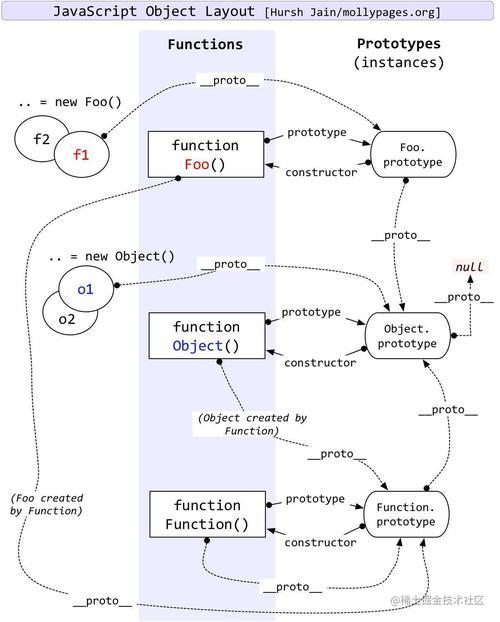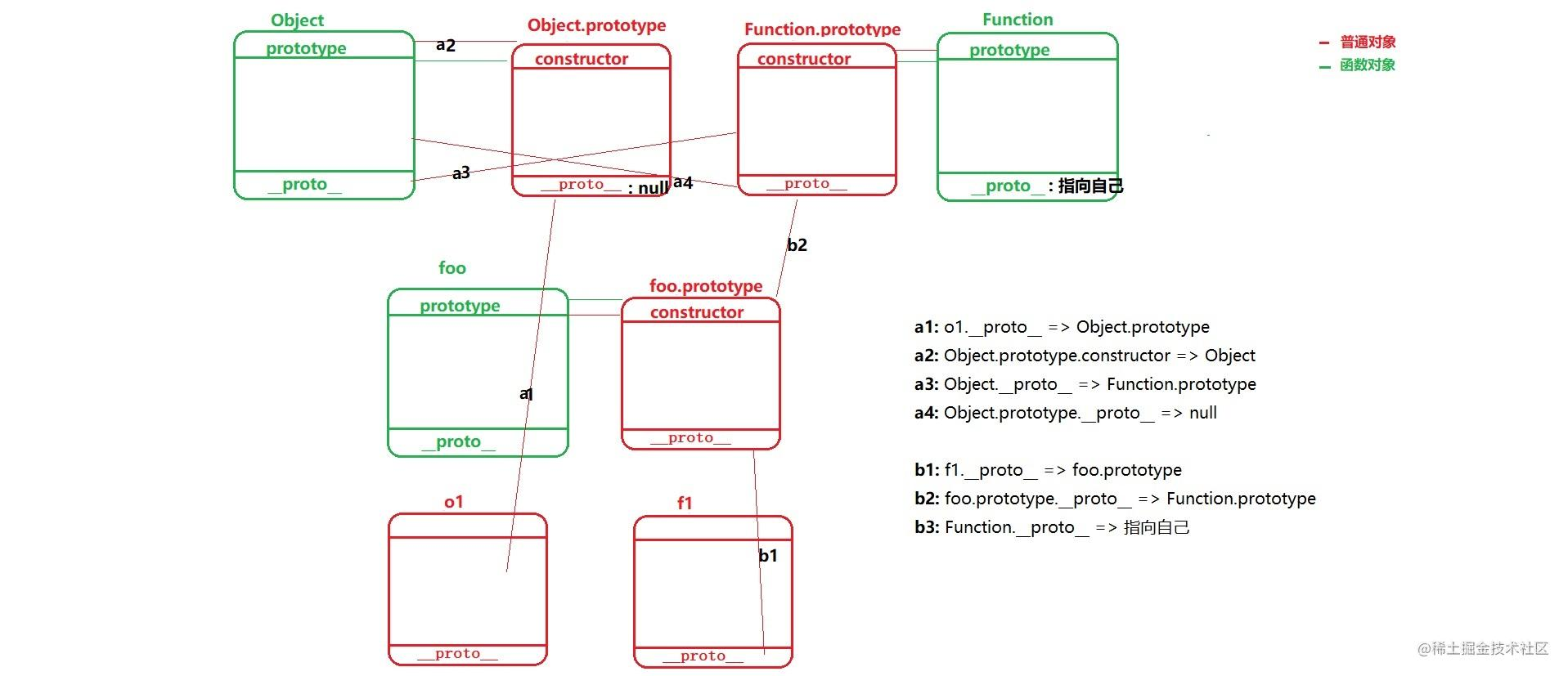• 实例对象为什么没有`prototype`: 只有构造函数才会有`prototype`属性指向原型对象
• `Object.prototype.__proto__`为什么是null: 原型对象(也就是浏览器为构造函数开辟的对象)都是Object基类的实例对象,所以原型对象的`__proto__`指向Object.prototype, 同样`Object.prototype.__proto__`也如此, 自己指向自己没有任何意义所以置为null
• `Function.__proto__`为什么指向自己: 上面说过内置`Function`也是通过`Function`构造出来的所以`Function.__proto__`也就指向了自己。

## 原型相关面试题

### 题一

``````class Re {
constructor () {
this.num = 3;
}
rand () {
return this.num;
}
}

var c1 = new Re();
console.log(c1.num, c1.rand());
Re.prototype.num = 4;
Re.prototype.rand = function () {
return this.num;
}
var c2 = new Re();
console.log(c1.num, c1.rand());
console.log(c2.num, c2.rand());

### 题二

``````let name = 'oop'
let Person = function (options){
this.name = options.name
}

Person.prototype.name = 'Person'
Person.prototype.getName = function(){
return this.name
}
let p = new Person({name: 'Beige'})

Object.getPrototypeOf(p) === p.__proto__;
let proto = Object.getPrototypeOf(p);
let targetObj = Object.assign(proto, {public: '前端自学驿站'})

console.log(targetObj === p.__proto__);
console.log(p.__proto__.constructor === Person)
console.log(p instanceof Person)
console.log(p.__proto__ === Person.prototype)
console.log(p.__proto__.public);
console.log(p.hasOwnProperty('name'))
console.log(p.hasOwnProperty('getName'))

let getName = p.getName
console.log(getName === Person.prototype.getName)
console.log(getName())
console.log(Person.prototype.getName())
console. log(p.getName())

### 题三

``````function Foo() {
getName = function () {console.log(1);};
return this;
}

Foo.getName = function() {console.log(2);};
Foo.prototype.getName = function() {console.log(3);};
var getName = function() {console.log(4);};
function getName() {console.log(5)}
Foo.getName();
getName();
Foo.getName();
getName();

var a = new Foo.getName();
var b = new Foo().getName();
var c = new new Foo().getName();
console.log(a, b, c);

``````function Foo() {
getName = function () {console.log(1);};
return this;
}
// 向Foo函数对象上添加私有成员
Foo.getName = function() {console.log(2);};
// 向函数原型上添加公有成员
Foo.prototype.getName = function() {console.log(3);};
// 表达式声明一个全局变量值为一个函数
var getName = function() {console.log(4);}; // 执行到这一步重新赋值了之前发声明式函数
// 声明一个函数： 优先级高于上面的方式
function getName() {console.log(5)}
Foo.getName(); // 2
getName(); // 4
Foo.getName(); // 2
getName(); // 4

// 难点
var a = new Foo.getName();
var b = new Foo().getName();
var c = new new Foo().getName();
console.log(a, b, c);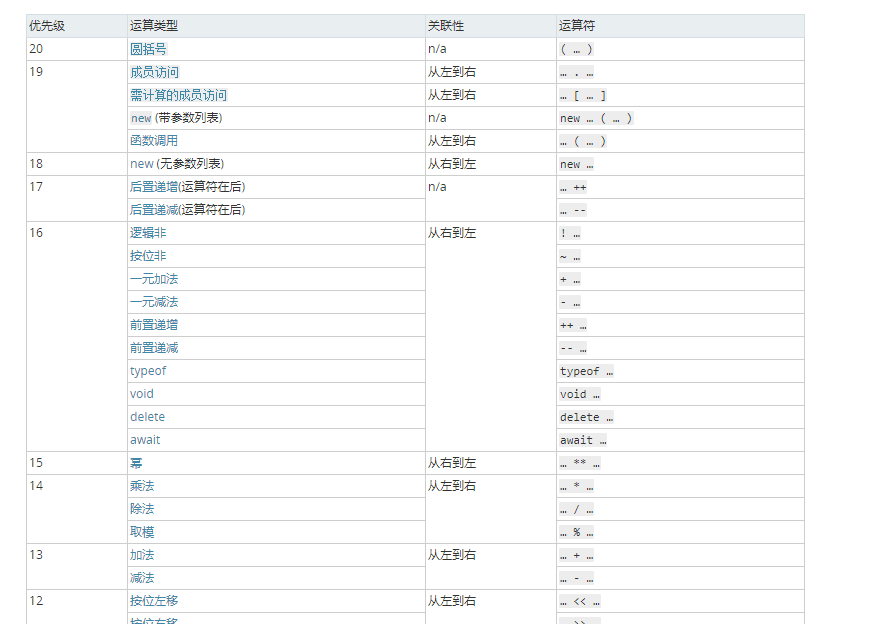``````var a = new Foo.getName();
// => 首先 new Foo.getName，按照优先级成员访问大于new不带括号，所以应该是这样
new (Foo.getName)() // => 将Foo.getName当做整体来new, 并不是有些人理解的 new (Foo.getName())

``````var b = new Foo().getName();
// 这个和上面就不一样了，new Foo()带了小括号和成员访问优先级一致，应该遵循从左到右
(new Foo()).getName()
// 返回的是new Foo()构造函数返回实例调用getName()方法的返回值

``````var c = new new Foo().getName();
// => new (new Foo().getName())
// => new (返回的实例.getName)()
// => 返回 new 实例.getName()构造出来的实例对象

``````// 我们稍微改下代码，验证上面是否正确
function Foo() {
getName = function () {console.log(1);};
return this;
}

Foo.getName = function(name) {
console.log(2);
this.name = name;
};
Foo.prototype.getName = function() {
console.log(3);
retrun '前端自学驿站'
// return {info: '自己返回的对象'} => 如果返回引用类型，第二三都会得到这个对象
};
var getName = function() {console.log(4);};
function getName() {console.log(5)}
Foo.getName();
getName();
Foo.getName();
getName();

var a = new Foo.getName(name);
// new (Foo.getName)() => 相当于将Foo.getName当做构造函数来new, 那我是不是可以传递参数
console.log(a) // {name: '北歌'}
console.log(a.__proto__.constructor === Foo.getName) // true

var b = new Foo().getName();
// (new Foo()).getName() => 最后返回的是实例调用.getName()方法返回的结果
// Foo并没有给实例添加私有的getName成员方法，所以调用的是原型上的方法，为了不影响下面的我返回非引用类型
console.log(b) // '前端自学驿站'

var c = new new Foo().getName();
// => new ( (new Foo())getName() ) // => 和上面的区别就是最后还new了下 实例.getName
console.log(c.__proto__.constructor === Foo.prototype.getName)
console.log(c) // {}

# 全面解析this

this:当前方法执行的主体(谁最后执行的这个方法,那么this就是谁,所以this和当前方法在哪创建的或者在哪执行的都没有必然的关系

## this的绑定规则及优先级

• 1.默认绑定（非严格模式情况下，this 指向 window, 严格模式下，this指向 undefined。）
• 2.隐式绑定（如果函数调用时，前面存在调用它的对象，那么this就会隐式绑定到这个对象上）
• 3.显式绑定（函数通过 call()、apply()、bind()调用，this 指向被绑定的对象。）
• 4.new 绑定（函数被 new 调用，this 指向由 new 新构造出来的这个对象。）

``````function test() {
this.a = 'Ge';
}

let obj = {
a: 'Bei',
fn() {
console.log(this);
}
};

function scope() {   // 放在函数里面防止影响结果
test() // 默认绑定
obj.fn() // 隐式绑定
test.call(obj) // 显示绑定
new test() // new 绑定
}

// 显示绑定
const Bar = test.bind(obj);

// new绑定
const bar = new Bar();
console.log(obj.a, '--', bar.a) // Bei -- Ge

new绑定改变了显示绑定中指定的this(obj)

## this的几种情况

• 全局作用域下的this指向window (严格模式也是)
• 如果给元素的事件行为绑定函数，那么函数中的this指向当前被绑定的那个元素
• 函数中的this，要看函数执行前有没有 (点) 有 (点) 的话，点前面是谁，this就指向谁，如果没有点，指向window 自执行函数中的this永远指向window
• 定时器中函数的this指向window
• 构造函数中的this指向当前的实例
• call、apply、bind可以改变函数的this指向
• 箭头函数中没有this，如果输出this，就会输出箭头函数定义时所在的作用域中的this
• 括号表达式也有可能改变this, 括号内只有一个项不会,两个或多个的话this指向window
1. 全局作用域中的this默认指向widnow，严格模式下没有就是undefined
``````function windowScope() {
// => this: window
}

2.给元素的某个事件绑定方法，方法中的this就是当前操作的元素本身

``````document.body.onclick = function () {
//=>this:body
};

3.函数执行，看函数前面是否有点，有的话，点前面是谁this就是谁，没有点，this是window（在JS的严格模式下，没有点this是undefined）

``````let fn = function () {
console.log(this.name);
};
let obj = {
name: '哈哈',
fn: fn
};
fn();//=>this:window
obj.fn();//=>this:obj

4.构造函数执行，方法中的this一般都是当前类的实例

``````let Fn = function () {
this.x = 100;//=>this:f
};
let f = new Fn;

5.箭头函数中没有自己的this,this是上下文中的this

``````let obj = {
fn: function () {
// this:obj
setTimeout(() => {
//this:obj
}, 1000);
}
};
obj.fn();

6.在小括号表达式中，会影响this的指向

``````let obj = {
fn: function () {
console.log(this);
}
};
(obj.fn)(); // => this:obj
;(12, obj.fn)();//=>this:window

``````function foo() {
console.log( this.a );
}

var a = 2;
var o = { a: 3, foo: foo };
var p = { a: 4};

o.foo(); // 3
(p.foo = o.foo)(); // 2
// 结论: 特殊情况的是如果括号表达式中有表达式语句执行, this也还是指向window
// 还有一种说法, p.foo = o.foo引用的内存地址, 也就是foo引用的内存地址, 匿名函数将foo引用的函数执行,
// this -> window

7.使用call/apply/bind可以改变this指向

``````fn.call(obj); // => this:obj
fn.call(12); // => this:12
fn.call(); // => this:window 非严格模式下call/apply/bind第一个参数不写或者写null和undefined，this都是window，严格模式下写谁this就是谁，不写是undefined

1. 定时器中的this指定window
``````setInterval(function() {
console.log(this);
}, 1000);

## this相关面试题

### 题一

``````var num = 10
const obj = {num: 20}
obj.fn = (function (num) {
this.num = num * 3
num++
return function (n) {
this.num += n
num++
console.log(num)
}
})(obj.num)
var fn = obj.fn
fn(5)
obj.fn(10)
console.log(num, obj.num)

### 题二

``````var a = {
name:"zhang",
sayName:function(){
console.log("this.name="+this.name);
}
};
var name = "ling";
function sayName(){
var sss = a.sayName;
sss(); //this.name = ?
a.sayName(); //this.name = ?
(a.sayName)(); //this.name = ?
(b = a.sayName)();//this.name = ?
}
sayName();

### 题三

``````var obj = {
a: 1,
foo: function (b) {
b = b || this.a
return function (c) {
console.log(this.a + b + c)
}
}
}
var a = 2
var obj2 = { a: 3 }

obj.foo(a).call(obj2, 1)
obj.foo.call(obj2)(1)

### 题四

``````var name = 'window'
function Person (name) {
this.name = name
this.obj = {
name: 'obj',
foo1: function () {
return function () {
console.log(this.name)
}
},
foo2: function () {
return () => {
console.log(this.name)
}
}
}
}
var person1 = new Person('person1')
var person2 = new Person('person2')

person1.obj.foo1()()
person1.obj.foo1.call(person2)()
person1.obj.foo1().call(person2)

person1.obj.foo2()()
person1.obj.foo2.call(person2)()
person1.obj.foo2().call(person2)

# 后语

## 系列文章

【前端体系】从一道面试题谈谈对EventLoop的理解 (更新了四道进阶题的解析)

【前端体系】从地基开始打造一座万丈高楼

【前端体系】正则在开发中的应用场景可不只是规则校验

## 参考文章

CONSTRUCTOR实现原理(转)

JavaScript的数据类型及其检测

JavaScript数据类型转换